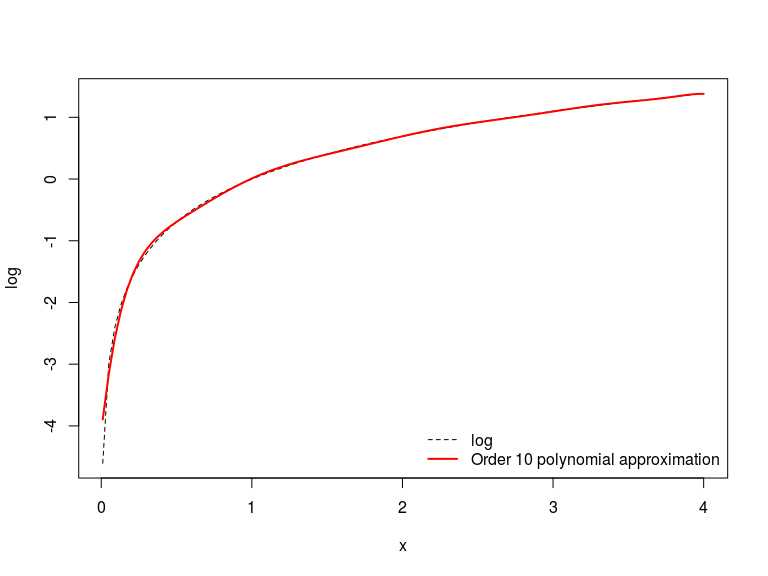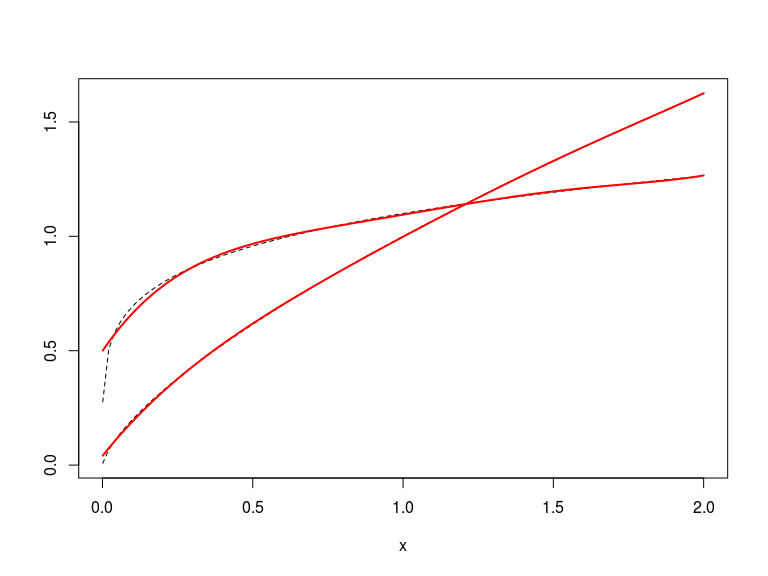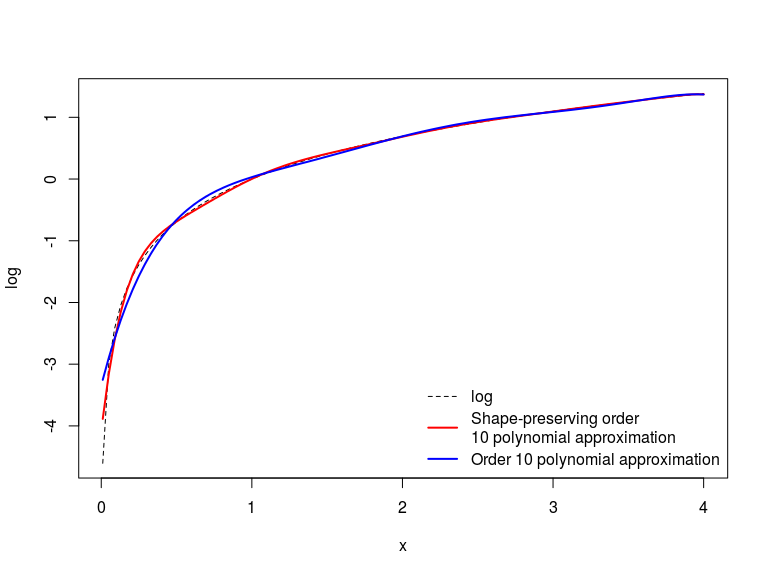# Introduction

The cheby package provides easy-to-use Chebychev interpolation of an arbitrary R function.

Chebychev interpolation approximates an arbitrary function on a closed interval using an the Chebychev polynomials as a basis. In general, polynomial approximation is helpful when a function f:[a,b]R$f:[a,b]\rightarrow \mathbb R$ is expensive to compute at all values in the domain. So instead, one approximates f()$f(\cdot)$ is by f^()$\hat f(\cdot)$, where:

f^(x)=i=1naiϕi(x)

Where ϕi(x)$\phi_i(x)$ are polynomials. In practice, this involves computing the function at a limited number of grid points, and then fitting a polynomial function through the function values at these points. This fitting is typically done by linear regression of the points on the fitting polynomials. This minimizes the square deviations at the grid points, but imposes no restrictions on the shape of the approximation.

If we want the resulting approximation to have particular properties (such as monotonicity or concavity), then we will need to use other methods. This package provides functionality to fit one-dimensional functions both via conventional Chebychev approximation, and by imposing constraints on the shape of the approximation.

The algorithms in this package draw heavily on Judd (1998).

# One-dimensional Chebychev approximation

## The Theory

Algorithm 6.2 of Judd (1998) outlines the steps required to produce the one dimensional Chebychev interpolation of order n$n$ on m$m$ nodes (with n<m$n) of the function f:[a,b]R$f:[a,b]\rightarrow \mathbb R$.

1. Compute the interpolation nodes on [1,1]$[-1,1]$ via:
zk=cos(2k12mπ)
2. Map the nodes to the interval [a,b]$[a,b]$
xk=(ba2)(zk+1)+a
3. Compute f()$f(\cdot)$ at the nodes
yk=f(xk),k=1,,m
4. Regress the yk$y_k$ on the Chebychev polynomials Ti(z)$T_i(z)$ for i=0,,n$i=0,\ldots,n$
ai=mk=1ykTi(zk)mk=1Ti(zk)2
5. The Chebychev approximation is then given by:
f^(x)=i=1naiTi(2(x1)ba1)

Theorem 6.7.3 of Judd (1998) shows that this approximation is pointwise-convergent for any Ck$C^k$ function.

## Basic approximation

The basic command for Chebychev interpolation is d1.poly. For example, to compute the approximation of the natural logarithm:

base <- d1.poly( fn=log, range=c(.01,4), iOrder=10, iPts=11 )

This computes a 6th-order approximation to log on the interval [0.01,4]$[0.01,4]$ (recall that log is not continuous at zero) using 7 grid points (the minimum possible). The output is a function that we can use straight away. We can check the approximation at a few points:

c( base(1), log(1) )
c( base(2), log(2) )
##  0.009866295 0.000000000
##  0.6928425 0.6931472

So the approximation is good to 2 decimal places for these values of x$x$. Better still, we can see the approximation visually:

plot( log, xlim=c(.01, 4), type='l', lty=2 )
plot( base, xlim=c(.01, 4), col='red', add=TRUE, lwd=2 )So the approximation is very good for most of the approximation range, although given the discontinuity at zero it struggles for small x$x$. And it is fast:

library(microbenchmark)
microbenchmark(base <- d1.poly( fn=log, range=c(.01,4), iOrder=6, iPts=7 ))
## Unit: milliseconds
##                                                                 expr
##  base <- d1.poly(fn = log, range = c(0.01, 4), iOrder = 6, iPts = 7)
##       min       lq   median       uq      max neval
##  10.71071 10.85061 11.45069 11.95571 15.90621   100

However, the approximation is not strictly concave - it oscillates slightly around the approximated function. Note how the dashed log function shows up alternately above and below the approximation. In some numerical calculations, preserving shape properties of the function can be important. Later we will discuss how value function will often fail if the continuation value function is not concave, as then there is no unique local interior maximum. This is not a problem which can be eliminated by simply increasing the order of the approximation, either. In fact this makes the problem worse. A higher order approximation improves the fit for small x$x$ but comes at the cost of more noticeable oscillations at large x$x$.

## Extracting more information about the approximation

To extract more information about the approximation, set the details flag to TRUE.

base.deets <- d1.poly( fn=log, range=c(.01,4), iOrder=10, iPts=11, details=TRUE )

This returns a five-element list with elements:

• fn The polynomial approximation function. So base.deetsfn(1)$fn(1)$ will equal base(1)$base(1)$
• poly The polynomial representation of the Chebychev approximation. This element is a polynomial from the polynom package, so can be manipulated easily (see the documentation of that package for more details). It is also defined only on the [1,1]$[-1,1]$ interval, so needs to be translated to the approximation interval to match the output of the fn element.
• fn.deriv The exact derivative of the polynomial approximation function. Computed by differentiating the polynomial representation and translating to the approximation interval. This is very useful for optimization problems where the derivatives are required. Plotting this function (not shown here) will generate upward-sloping parts of the function, confirming that the approximation is not globally concave. We can check also this calculation by comparing to the finite-difference calculation:
1e06 * ( base(1+1e-06) - base(1) )
base.deets$fn.deriv(1) ##  0.76435 ##  1.112838 • fn.deriv.poly The polynomial representation of the derivative. The analogue of poly, but for deriv. • residuals The residuals of the approximation. All zero if iPts is one more than iOrder. ## Pre-computing function values The “standard” usage of d1.poly above integrates function calculation and approximation. This is convenient - it simply allows one to pass a function to the approximation and get a function back. But it is not very flexible - it requires a self-contained function to be evaluated separately at each of the points in the grid. In many applications this is not efficient. For example, if the target function to be evaluated contains a computationally demanding common component, it may be quicker to calculate that on its own and then include that value in the evaluation of f()$f(\cdot)$ at each of the grid points. Here is an example of how to use this syntax grid.pts <- d1.grid( c( .01, 4), 11 ) # The grid of Chebychev nodes log.vals <- sapply( grid.pts, log ) # The target function evaluated at the approximation nodes base.grid <- d1.poly( fn.vals=log.vals, grid=grid.pts, range=c(.01, 4), iOrder=10, iPts=11, details=TRUE ) The inclusion of the argument grid here is superfluous, as without this d1.poly assumes that the function values are evaluated on a grid of Chebychev nodes, which is exactly what d1.grid produces here. If you wish to evaluate the function on a different grid of nodes, you should use this argument to specify that grid. ## Passing function parameters One can pass function parameters using the argument fn.opts. However, this requires that the target function have all its parameters enter through a single argument named opts (using a list to pass multiple parameters where required). For example, to compute a fifth-order approximation to the function Akα$A k ^ \alpha$ where A$A$ and α$\alpha$ are parameters: target <- function( k, opts ) return( opts$A  * k ^ opts\$alpha )
# The target function
apx.1 <- d1.poly( target, c(.001,2), 5, 6, fn.opts=list(A=1, alpha=.7) )
apx.2 <- d1.poly( target, c(.001,2), 5, 6, fn.opts=list(A=1.1, alpha=.2) )
# The approximations
plot( function(x) target(x, opts=list(A=1, alpha=.7) ), xlim=c(.001,2), lty=2, ylab='' )
plot( function(x) target(x, opts=list(A=1.1, alpha=.2) ), xlim=c(.001,2), lty=2, add=TRUE )
plot( apx.1, xlim=c(.001,2), col='red', lwd=2, add=TRUE )
plot( apx.2, xlim=c(.001,2), col='red', lwd=2, add=TRUE )## Approximating mulit-variable functions

Functions of more variables can be approximated using dn.poly. This works much the same as d1.poly1, but currently only works for functions of two variables.

# One-dimensional shape-preserving Chebychev approximation

## The theory

For details of the theory, see Judd section 6.11. The basic idea of shape-preserving Chebychev approximation is to minimize the (squared) errors of a polynomial fit on an approximation grid, subject to constraints on the shape. This task is therefore a constrained optimization problem where the objective is the error on the approximation, the controls are the polynomial coefficients, and the constraints are on the shape of the polynomial at a secondary grid (typically expressed as restrictions on the sums of the polynomial coefficients).

## Usage

To approximate a univariate function using a shape-preserving polynomial, we can use sp1.poly. This function takes the same inputs as d1.poly, but now also requires details on the shape-preserving restrictions on the polynomial approximation. These details are provided through the arguments n.shape and sign.deriv. The former is a vector establishing the number of Chebychev nodes at which shape restrictions should be imposed. The second is a vector of positive and negative unit values determining the sign of the restriction. For example, to restrict the approximation to be increasing at 3 points and concave at 7, one would use set n.shape=c(3,7) and sign.deriv=c(1,-1).

Here’s a complete example:

base.sp <- sp1.poly( fn=log, range=c(.01,4), iOrder=10, iPts=11, n.shape=c(3,21), sign.deriv=c(1,-1) )
## Sucess: nlopt terminated after 63 iterations
## NLOPT_XTOL_REACHED: Optimization stopped because xtol_rel or xtol_abs (above) was reached.
plot( log, xlim=c(.01,4), lty=2 )
plot( base.sp, xlim=c(.01,4), add=TRUE, lwd=2, col='red' )
plot( base, xlim=c(.01,4), add=TRUE, lwd=2, col='blue' )`Here, we impose concavity at 21 points in the approximation interval and monotonicity at only 3 (as the standard Chebychev approximation delivers near-monotonicity already). The plot above show that this generates concavity for small values of x$x$, where the standard approximation oscillates much more. The cost, of course, is a poorer fit of the approximation near zero.

Shape-preserving polynomial approximation is particularly useful when the true function has known properties that influence subsequent calculations. For example, imagine that f(x)$f(x)$ is very hard to compute and we want to find the maximum (e.g. a complicated likelihood, or a value function in dynamic programming). If f(x)$f(x)$ can be shown to be concave then it has a unique maximum. But if the approximationg function f~(x)$\tilde f(x)$ is not concave, then its maximum will not be unique. This can introduce large errors even if f~(x)$\tilde f(x)$ and f(x)$f(x)$ are very close in a pointwise sense.